Applied and Computational Mathematics
Volume 4, Issue 6, December 2015, Pages: 462-468

Reliability Based Optimization with Metaheuristic Algorithms and Latin Hypercube Sampling Based Surrogate Models

Liu Chu*, Eduardo Souza De Cursi, Abdelkhalak El Hami, Mohamed Eid

Laboratory of Optimization and Reliability in Mechanical Structure, Department of Mechanics, National Institute of Applied Science of Rouen, France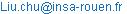(L. Chu)

Liu Chu, Eduardo Souza De Cursi, Abdelkhalak El Hami, Mohamed Eid. Reliability Based Optimization with Metaheuristic Algorithms and Latin Hypercube Sampling Based Surrogate Models. Applied and Computational Mathematics. Vol. 4, No. 6, 2015, pp. 462-468. doi: 10.11648/j.acm.20150406.20

Abstract: Reliability based optimization (RBO) is one of the most appropriate methods for structural design under uncertainties. It searches for the best compromise between cost and safety while considering system uncertainties by incorporating reliability measures within the optimization. Despite the advantages of RBO, its application to practical engineering problem is still quite challenging. In this paper, we propose an effective method to decouple the loops of reliability assessment analysis and optimization by creating surrogate models. The Latin Hypercube sampling approach is applied to a structural finite element model to obtain an effective database for building surrogate models. In order to avoid premature convergence of the optimization process, the RBO problem is solved with metaheuristic methods such as genetic algorithm and simulated annealing. The relative efficiency of surrogate models and their relationship with metaheuristic search engine are discussed in the article.

Keywords: Topology and Sizing Optimization of Trusses, Gravitational Search Algorithm, Efficient Member Grouping, Double and Triple Layer Grid Structures

1. Introduction

Uncertainty is an inevitable issue in the process of manufacture, infrastructure, and engineering design. Quantifying and propagating the uncertainty in the simulation or design process as a key component of risk analysis, robustness evaluation or reliability based optimization (RBO) attracts attention of researchers and designer . Among numerous methods of uncertainty propagation analysis, the most commonly used method is Monte Carlo Simulation . It is a non-intrusive, sampling based numerical method, but often requires a large ensemble of sampling points to provide a reliable and stable estimate of uncertainty. This makes MCS computationally expensive. However, using an appropriated sampling strategy (for example, weighted sampling, first-order sensitivity method, Latin hypercube sampling - LHS) allows the member of MCS sampling points to be significantly reduced yet reaching the target level of accuracy . The LHS method will be selected in this research as MCS sampling procedure in view of its capability to cover the whole range of each sampled variable.

The reliability analysis of complex and realistic structural systems is in general a computationally demanding task. Several surrogate models suitable for structural reliability design are presently available in the literature . The first option is to apply first- and second- order polynomial regression models as surrogates for the true limit state function. More recently, attention has been given to alternative approaches based on artificial neural networks, support vector machines and Kriging interpolation models . When compared with the polynomial regression models, Kriging interpolation models for structural reliability problems have several competitive features. Besides interpolation capability, Kriging models have enough flexibility to approximate arbitrary functions with a high level of accuracy, and can assess the level of uncertainty of model predictions .

Traditional methods may suffer from the unaffordable computational costs of global optimization or premature convergence to local optima . This study attempts to solve the RBO problem with two well established metaheuristic methods such as Genetic Algorithm (GA) and Simulated Annealing (SA).

GA draws inspiration from the principles and mechanisms of natural selection  to perform search and optimization. This technique falls in the more general category of evolutionary algorithms. SA mimics the annealing process used in the metallurgical industry, by which slow cooling is applied to metals to produce better aligned, low energy-state crystallization . SA is a local search-based algorithm able to bypass local optima because it searches not only for trial solutions that may reduce the cost function, but also allows moves leading to increase the objective function value. This mechanism may avoid the optimization search being trapped prematurely in a local minimum .

Besides SA and GA, there are other metaheuristic methods such as Neural Networks (NN) and Support Vector Machines (SVM). NN are inspired by biological neuron systems, but they do not always work well as they suffer greatly from problems of under-fitting and over-fitting . SVM extends the ideas of Neural Networks. Therefore, SA and GA seem more appropriate than NN and SWM for the purpose of this study.

The paper presents an effective method to decouple the reliability assessment analysis and optimization stages in RBO problems. For that purpose, LHS is utilized to build a reliable database for approximating the response of a structural finite element model; sensitivity analysis is carried out to obtain the number of samples yielding a good compromise between model accuracy and computational cost. Once the database is defined, we compare the relative performance of three approximation methods to fit the probabilistic response of the structural FE model: namely, response surface method (first order and second order polynomial regressions), nonlinear fitting method and Kriging models. Then, a reliability based optimization problem where the objective is to minimize the weight of the structure and minimize the probability of failure is solved with GA and SA.

In view of this, the paper will be structured as follows. Section 2 describes the sensitivity analysis carried out in purpose of selecting the optimal size of LHS. Section 3 compares the above mentioned data fitting methods. The RBO problem is described in Section 4 and optimization results also are discussed in this section. Finally, Section 5 summarizes the main finding of the study.

2. Latin Hypercube Sampling for the Structural Finite Element Model

Monte Carlo simulation (MCS) methods (sampling-based methods) perform repeated sampling and simulation. It is useful if one is trying to get a model to imitate a random sampling from a population or for doing statistical experiments. It provides  the most effective approach to the propagation and analysis of uncertainty for a number of reasons. First, the sampling based approach covers the full range of each uncertain variable in a complicated system. Second, modification of the model is not required, and direct estimates of distribution functions are provided. In addition, in the process of sampling, a variety of sensitivity analysis procedures are available. Last but not the least, analysis procedures can be developed and allow the propagation of results through systems of linked models .

However, MCS can keep a certain level of accuracy only if a very large number of iterations are performed. It is obvious that MCS methods become computationally prohibitive when simulation model is complex. To be more efficient than the random sampling method, several improved MCS methods with different sampling techniques have been developed. Importance sampling (weighted sampling), is expected to reduce error to zero if the probability density function is properly selected . The first-order sensitivity method, as a variance reduction technique, is also utilized to accelerate MCS estimation convergence . The variance reduction techniques are especially important when MCS is applied to estimate small failure probability .

The LHS approach is an effective way to improve computational efficiency of MCS methods. It divides the range of each variable into disjoint intervals of equal probability, and one value is randomly selected from each interval . This allows MCS stability to be improved and preserves tractability of random sampling.

In order to propagate uncertainty into a structural finite element model, we first created a deterministic FEM with ANSYS commercial software: the cylindrical thin shell shown in Fig.1. Geometric parameters and material parameters are listed in Table1. Besides deterministic parameters, there are also indicated on the displacements and deformations of the structure (see Fig.1). Here, we are interested in the maximum stress generated in the structure in each sampling loop.

The input variables of LHS method were the probabilistic parameters described in Table 1 while the output parameter was the maximum stress of the structure. In order to check the stability of LHS, different numbers of samples were attempted in this study. Figure 2 shows the scattered results of LHS computations. Cumulative probability and probability density distribution for the maximum stress selected as output parameter are plotted in Fig.3 and 4, respectively. It can be seen that the method is rather insensitive to the number of samples; hence, LHS is very stable and has good convergence. A more detailed analysis of results reveals that the peak value of the probability density of maximum stress increases with the number of samples. A good compromise between accuracy and computational cost was achieved by setting the number of samples equal to 500.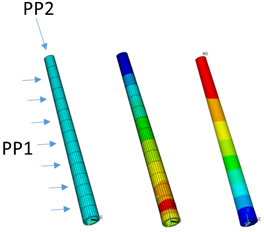Fig. 1. Finite element model and results of structural analysis.

Table 1. Parameters of material and geometrical properties.

 Material and geometrical properties Certain parameters L Length of the structure 10 m E Young modulus 2*10^5 MPa P Physical density 7000 kg/m^3 V Poisson ratio 0.3 Variables in Latin Hypercube sampling methods R1 Radius in the bottom 0.1 ~3 m R2 Radius in the top 0.1~3 m T1 Ratio of ring thickness 0.01~0.99 PP1 Pressure in the half surface 0~240 MPa PP2 Force in the top 0~10^5 N

In Latin Hypercube sampling method, the input variables were parameters described in Table 1. The output parameter was the maximum stress of the structure in each certain iteration of mechanical analysis. To test the stability of Latin Hypercube sampling method, different numbers of samples were attempted in our program. As presented in Fig. 2, scatter results of Latin Hypercube sampling.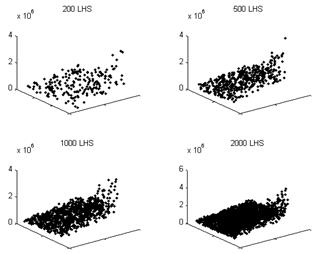Fig. 2. Sampling points of LHS.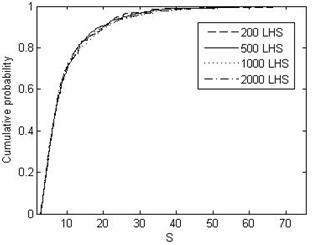Fig. 3. Cumulative probability of maximum stress (MPa).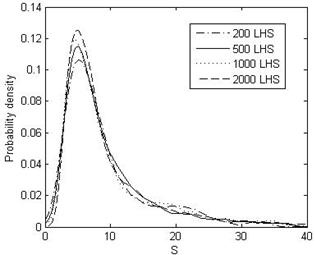Fig. 4. Probability density of maximum stress (MPa).

3. Surrogate Models of Structural Response

A surrogate model fits a set of data, input-output pairs obtained by evaluating a black-box model of a complex system . Here, the black-box model is the Latin Hypercube sampling performed in Finite Element Model. Surrogate models may be classified in two categories based on their purpose. For example, the Response Surface Method (RSM) can be applied in a sequential manner to approximate response in small regions of the design space that change as the optimization process converges to the optimum. First and second order polynomial RSM models can be expressed as :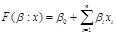(1)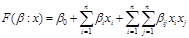(2)

Global surrogate models approximate the system’s response over the whole design space. In particular, Kriging models fit a spatial correlation function to a data set consisting of input-output pairs. That is: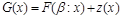(3)

Where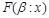is a deterministic component defined by a regression model that gives an approximation to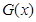in mean value and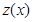is a stationary Gaussian process with zero mean and covariance ,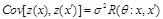(4)

That interpolates the errors between the regression model predictionsand the true limit state function valuesat the m base points;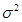the constant process variance and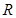is a prescribed correlation function.

Several correlation functions (e.g. exponential, linear and Gaussian) are available. The most widely used correlation function in structural reliability problems is the anisotropic Gaussian correlation function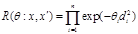(5)

With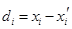is the distance between the currently trial point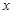and the base point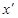in the ith dimention of space of random design variables and the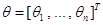vector defines the inverse of correlation lengths in each direction.

A kriging interpolation model is completely defined by a vector of regression coefficients, a vector of correlation parameterand the varianceof the stationary Gaussian process. These parameters are estimated by fitting the Kriging model over a set of support points.

Thevector has to be first estimated using the method of maximum likelihood: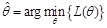(6)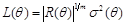(7)

Its prediction at a given point of the space of basic random variables can be obtained,(8)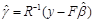(9)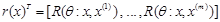(10)

Where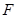is the regression matrix and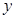is the vector of true values of the function to be approximated. The matrixdefines the correlation between each pair of support points according to the prescribed correlation function.

The following vector including correlations between the currently examined trial point and the m base points used for fitting the Kriging model represents the expected or mean value predicted by the Kriging model. The variance or uncertainty associated with Kriging model predictions can be estimated as: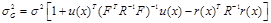(11)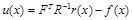(12)

In this study, surrogate models were created using first and second order response surfaces described by Eqs. (1,2), the Kriging model described by Eq.(3), and the general nonlinear fitting given in Eq.(13).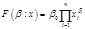(13)

The correlation coefficient and average error between predicted values and true values were computed for all fitting method to assess their relative performance. For this purpose, the following equations were utilized: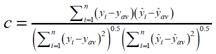(14)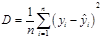(15)

Table 2. Comparison of results of surrogate model.

 Correlation coefficient D Non-linear fitting 0.9933 1.0585 1-order regression 0.8002 23.7895 2-order regression 0.9471 6.8092 KM(0-order) 1 5.9341e-17 KM(1-order) 1 1.6060e-19 KM(2-order) 1 3.5514e-21

Tabel 2 shows that response surface method could not efficiently fit the huge database generated by the LHS random sampling. In fact, correlation coefficient is far from 1 and average errors also are very large. Since nonlinear fitting is slightly more efficient than RSM, it was chosen as comparison basis with the Kriging models which are definitely the best fitting approach. In addition, the correlation of Kriging models was 1 regardless of the model order. For the test problem considered in this study, the lowest average error was obtained using the second order Kriging model.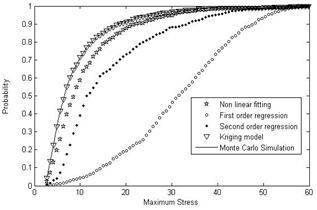Fig. 5. Stress (MPa) cumulative probability trends predicted by surrogate models compared in this study.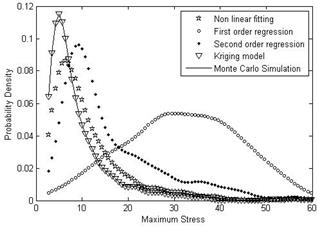Fig. 6. Stress (MPa) probability distributions predicted by surrogate models compared in this study.

Predictions of surrogate models on cumulative probability and probability density are respectively shown in Figs. 5 and 6 for all fitting methods. Since results of all Kriging models practically coincided, plots refer to the first order model. It can be seen that Kriging model approximates very well probability distributions and cumulative probability trends for the whole design space. Nonlinear fitting is the second best model while response surface method does not fit efficiently the original database. In view of this, Kriging model was selected to approximate the functional relationship between design variables and structural response that allows FE analysis to be avoided. Its advantage in RBO is discussed in the next section.

4. Reliability Based Optimization with Surrogate Models

The section describes the reliability based optimization algorithm including surrogate models utilized in this study. The surrogate models discussed in Section 3 are summarized by the flowchart shown in Fig.7.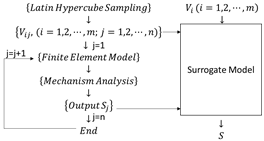Fig. 7. Flowchart of surrogate models for structural analysis.

RBO accounts for inherent randomness in physical quantities, such as element dimensions, material properties and external loads. It can be divided into three categories: two-level methods, single loop methods and decoupled methods . In two-level methods, reliability analysis and optimization are two nested loops. Reliability index approach (RIA) and the performance measure approach (PMA) are widely used for reliability analysis . However, convergence problems may arise if concave performance measure functions are involved. Single loop RBO methods transform nested optimization into a single loop process by replacing reliability constraints with the Karush-Kuhn-Tucker optimality conditions . This approach requires explicit implementation of the probabilistic transformation and calculation of the second order derivatives.

Decoupling methods transform the RBO problem into a deterministic one by approximating the probability of failure as a function of the design variables . A way to build such an approximation is to adopt a predefined function and select some predefined interpolation points in the design space. The Kriging model was chosen in this study for that purpose. Based on the sampling points extracted from the LHS method applied to the FE model, it is possible to construct the Kriging model which approximately describes the relationship between the design variables and the structural response on stress. The optimization problem can be stated as follows:

Minimum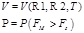Subject to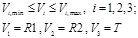The objective of optimization is hence to minimize the structural weight and the probability of failure. In particular,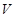is the volume of the structure that depends linearly on weight /cost of the structure. The bounds of design variables, the minimum and the maximum were selected from practical considerations.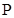is equal to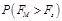, it is the probability that the maximum stress developed in the structure is higher than the yield limit. Load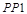and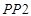were already defined in Table 1. In order to simulate uncertainties in working conditions, specific probability distribution functions were chosen and set equal to certain values to reproduce a practical scenario.

The optimization problem was solved with genetic algorithm (GA) and simulated annealing (SA). GA optimization starts from a random initial population: each individual (chromosome) is encoded into a structure representing its properties and evolves through successive generations. In each generation, chromosomes are rated with respect to their fitness.

Table 3. Results of RBO.

 GA(KM) GA(NF) SA(KM) SA(NF) R1 1.1879 0.3532 1.1063 0.3399 R2 0.1092 0.1175 0.1022 0.1001 T 0.9887 0.9896 0.9872 0.9900 P 0.0100 0 0.0320 0 V 0.0349 0.0037 0.0342 0.0032 Time 2619 1.104 2244 4.436

The statement of the RBO problem is obviously the same for SA. However, a new solution is generated in the neighborhood of the current solution. The trial design is compared with the current best solution and replaces it if the cost function is smaller. A temporary increase in cost function is tolerated based on a threshold probability that it will lead to improve design in the next iterations. GA and SA search continues until some stopping criterion is met (for example, maximum number of analyses). Table.3 shows the RBO results obtained by GA and SA converged practically to the same design. However, the computational cost of the optimization is much smaller in the case of nonlinear fitting.

The optimum design must be evaluated with respect to propagation of uncertainty. Fig. 8 and 9 show the results of LHS performed with respect to the optimum design. It appears that nonlinear fitting surrogate model does not predict correctly the maximum stress in the structure: therefore, there is a problem with distortion in prediction. Furthermore, the probability of failure is much larger than the optimized value that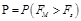equal to zero. In view of this, we might conclude that nonlinear fitting surrogate model is not suited for reliability based optimization. SA converged to a better design but required a much higher computation cost than GA. Kriging model was definitely more efficient than nonlinear fitting as surrogate model. Furthermore, the optimum designs found by SA and GA could satisfy the safety criteria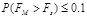also when uncertainty is propagated into the model. SA performed slightly better than GA saving more material and achieving higher structural reliability. Furthermore, SA was about 15% faster than GA.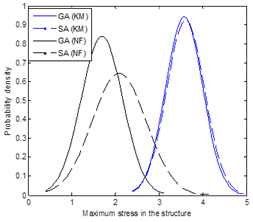Fig. 8. Stress (MPa) probability distributions predicted for optimized designs.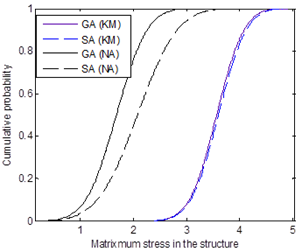Fig. 9. Stress (MPa) cumulative probability trends predicted for optimized designs.

5. Conclusion

The paper proposed a method to decouple the loops of reliability assessment analysis and optimization in RBO problems. The basic idea is to create reliable surrogate models using the Latin Hypercube sampling. A simple RBO problem where the objective was to minimize structural weight and probability of failure was solved with genetic algorithm and simulated annealing. The Kriging model was found to be considerably more efficient than response surface and nonlinear fitting methods. The proposed optimization framework successfully solved the RBO test problem considered in this research.

References

1. D.L. Phillips, M.Spatial. Uncertainty analysis: propagation of interpolation errors in spatially distributed models. Ecological Modelling, Volume 91, Issues 1–3, 15 November 1996.
2. P Doubilet, CB Begg, MC Weinstein. Probabilistic sensitivity analysis using Monte Carlo simulation. A practical approach.Journal of the Society for Medical Decision Making [1985, 5(2):157-177].
3. J.C. Helton, F.J. Davis. Latin hypercube sampling and the propagation of uncertainty in analyses of complex systems. Reliability Engineering & System Safety, 2003.
4. John C. Brigham, W. Aquino. Surrogate-Model Accelerated Random Search algorithm for global optimization with applications to inverse material identification.Computer Methods in Applied Mechanics and Engineering, Volume 196.
5. J.-C. Jouhaud, P. Sagaut, M. Montagnac, J. Laurenceau.A surrogate-model based multidisciplinary shape optimization method with application to a 2D subsonic airfoil.Computers & Fluids, Volume 36, Issue 3, March 2007, Pages 520-529.
6. Jack P.C. Kleijnen. Kriging metamodeling in simulation: A review. European Journal of Operational Research, Volume 192, Issue 3, 1 February 2009, Pages 707-716.
7. H.N. A. Pham, E. Triantaphyllou. A metaheuristic approach for improving the accuracy in some classification algorithms. Computers & Operations Research, Volume 38, Issue 1, January 2011, Pages 174-189.
8. A. F. Shahraki, R. Noorossana. Reliability-based robust design optimization: A general methodology using genetic algorithm. Computers & Industrial Engineering, Volume 74, August 2014, Pages 199-207.
9. P. S. Laursen. Simulated annealing for the QAP — Optimal tradeoff between simulation time and solution quality. European Journal of Operational Research, Volume 69, Issue 2, 10 September 1993, Pages 238-243.
10. J. R. Harland, P. Salamon. Simulated annealing: A review of the thermodynamic approach. Nuclear Physics B - Proceedings Supplements, Volume 5, Issue 1, September 1988, Pages 109-115.
11. H. Ishigami, T. Fukuda, T. Shibata, F. Arai. Structure optimization of fuzzy neural network by genetic algorithm. Fuzzy Sets and Systems, Volume 71, Issue 3, 12 May 1995, Pages 257-264.
12. B.G.J. Thompson, B. Sagar. The development and application of integrated procedures for post-closure assessment, based upon Monte Carlo simulation: the probabilistic systems assessment (PSA) approach. Reliability Engineering & System Safety, Volume 42, Issues 2–3, 1993, Pages 125-160.
13. L. Goel, R. Billinton. Monte Carlo simulation applied to distribution feeder reliability evaluation. Electric Power Systems Research, Volume 29, Issue 3, May 1994, Pages 193-202.
14. R.E. Melchers. Importance sampling in structural systems.Structural Safety, Volume 6, Issue 1, July 1989, Pages 3-10.
15. M. Z. Miguel, G. Josselin, R.Emmanuel.An original sensitivity statistic within a new adaptive accelerated Monte-Carlo method.Procedia - Social and Behavioral Sciences, Volume 2, Issue 6, 2010, Pages 7712-7713.
16. Y. Liu, M. Y. Hussaini, G.Ökten. Optimization of a Monte Carlo variance reduction method based on sensitivity derivatives.Applied Numerical Mathematics, Volume 72, October 2013, Pages 160-171.
17. A. Florian. An efficient sampling scheme: Updated Latin Hypercube Sampling. Probabilistic Engineering Mechanics, Volume 7, Issue 2, 1992, Pages 123-130.
18. B. Gaspar, A.P. Teixeira, C. G. Soares.Assessment of the efficiency of Kriging surrogate models for structural reliability analysis. Probabilistic Engineering Mechanics, Volume 37, July 2014, Pages 24-34.
19. M. Miki, Y. Murotsu, T. Tanaka, S. Shao. Reliability-based optimization of fibrous laminated composites. Reliability Engineering & System Safety, Volume 56, Issue 3, June 1997, Pages 285-290.
20. A. D. Kiureghian, T. Dakessian. Multiple design points in first and second-order reliability. Structural Safety, Volume 20, Issue 1, 1998, Pages 37-49.
21. S. Shan, G. Wang. Reliable design space and complete single-loop reliability-based design optimization. Reliability Engineering & System Safety, Volume 93, Issue 8, August 2008, Pages 1218-1230.
22. G. I. Schuëller, H. A. Jensen. Computational methods in optimization considering uncertainties – An overview. Computer Methods in Applied Mechanics and Engineering, Volume 198, Issue 1, 15 November 2008, Pages 2-13.

 Contents 1. 2. 3. 4. 5.
Article ToolsAbstractPDF(2497K)